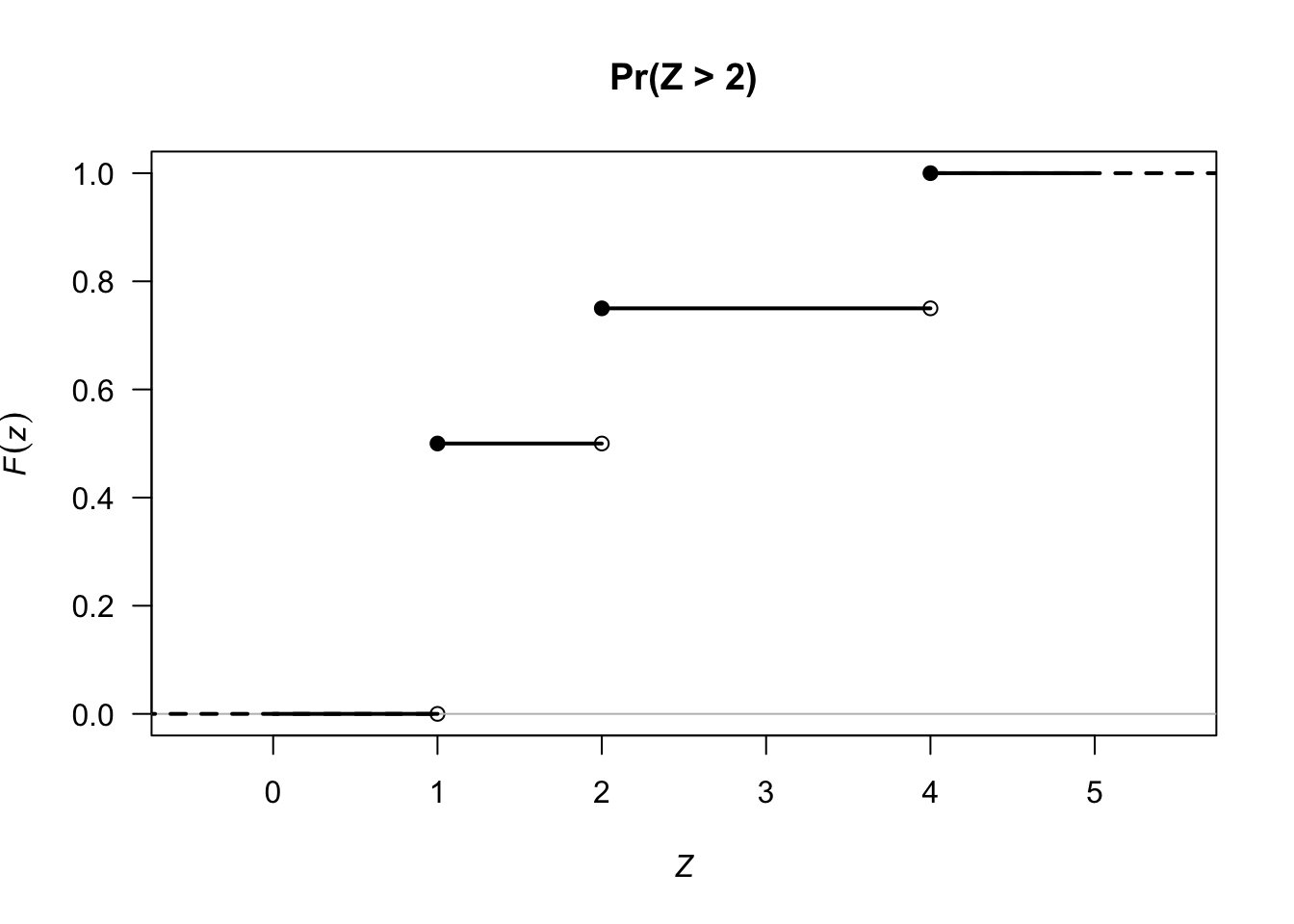## 3.2 CDF for discrete rvs

The CDF $$F_X(x)$$ for a discrete rv $$X$$ with PMF $$f_X(x)$$ is $F_X(x) = \Pr(T \le x) = \sum_{-\infty}^x f(t).$

Example 2.10 (CDF: discrete rv) Consider the discrete rv $$Z$$ from Example 2.6, with PMF $f_Z(z) = \frac{|z - 3|}{4} \quad\text{for z=1, 2, 3, 4}.$ The CDF is
$F_Z(z) = \left\{ \begin{array}{ll} 0 & \text{for z<1}\\ 1/2 & \text{for 1\le z < 2}\\ 3/4 & \text{for 2\le z < 4}\\ 1 & \text{for z\ge4}. \end{array} \right.$ See Fig. 3.2.FIGURE 3.2: The cumulative distribution function (CDF) for $$Z$$. Open dots mean that the point IS NOT included; solid dots mean the point IS included